# P5018对称二叉树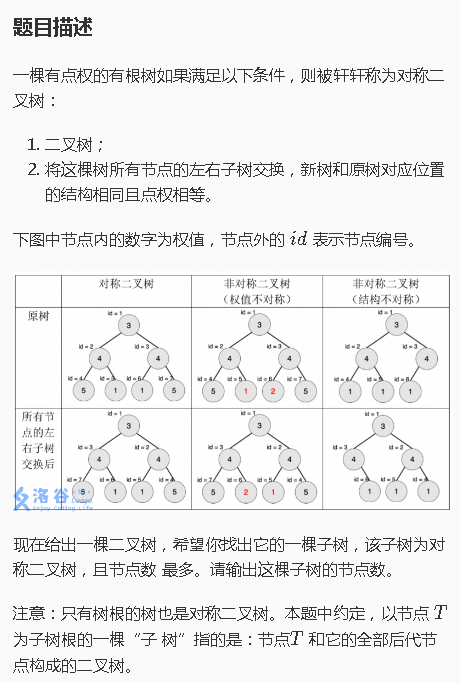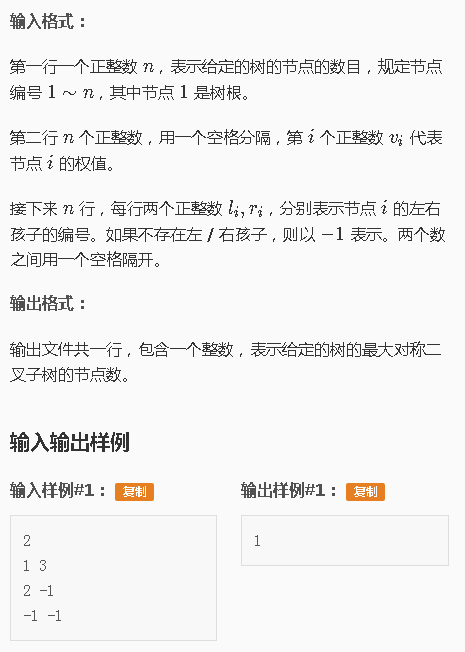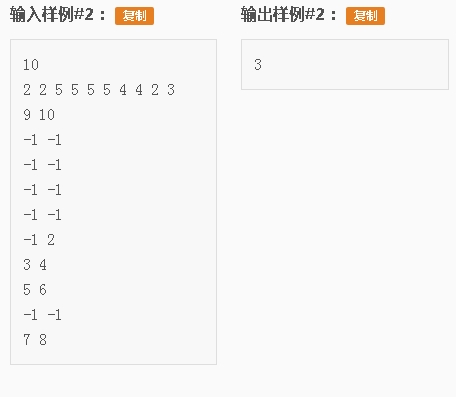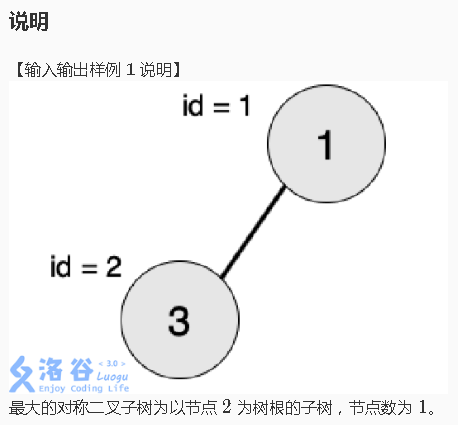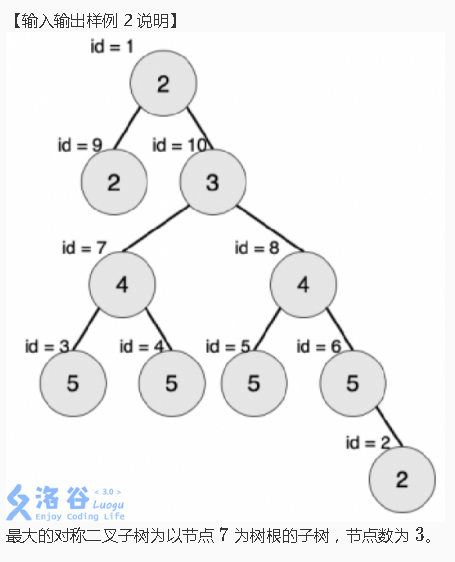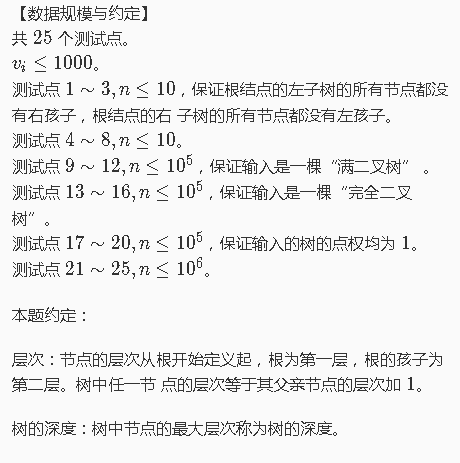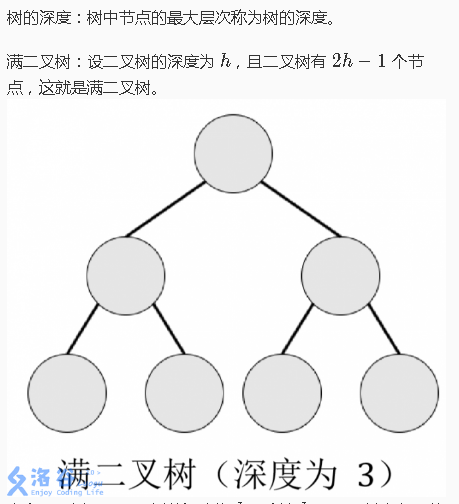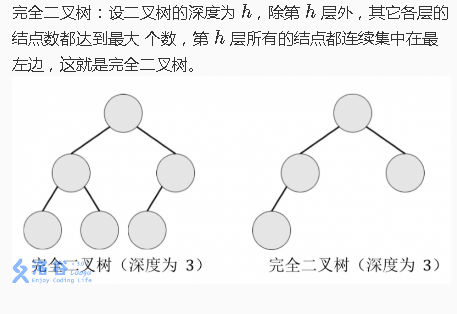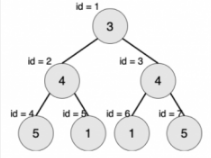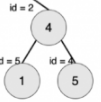这并不是对称二叉树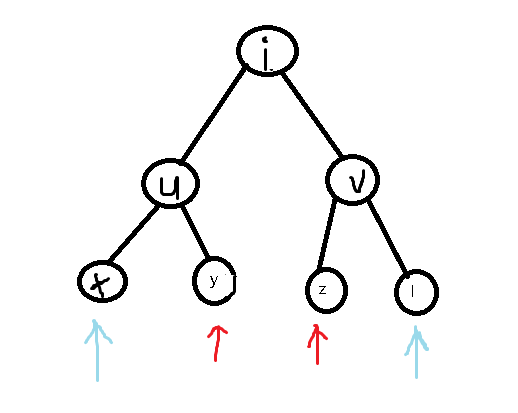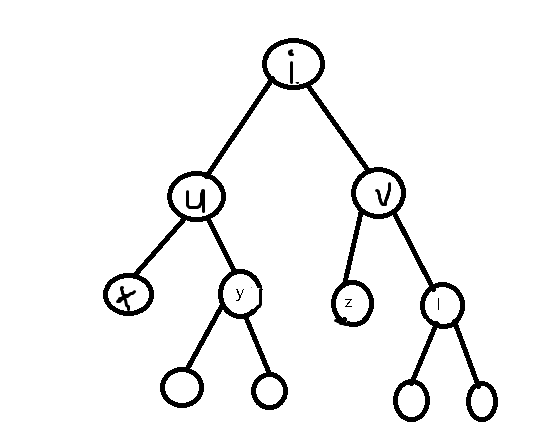%%%wxl用十几分钟讲完了这道题

#include <bits/stdc++.h>
using namespace std;
int n,son,node,size;
{
char ch=getchar();
int x=0;bool f=0;
while(ch<'0'||ch>'9')
{
if(ch=='-')f=1;
ch=getchar();
}
while(ch>='0'&&ch<='9')
{
x=(x<<3)+(x<<1)+(ch^48);
ch=getchar();
}
f?x=-x:x=x;
return x;
}
void dfs(int x)//预处理子树大小(size)
{
size[x]=1;
if(son[x]!=-1)
{
dfs(son[x]);
size[x]+=size[son[x]];
}
if(son[x]!=-1)
{
dfs(son[x]);
size[x]+=size[son[x]];
}
}
bool check(int u,int v)//一个长成check的搜索
{
if(u==-1&&v==-1)return true;//注意如果左右儿子都不存在，那这是个叶节点，也是对称二叉树
if(size[u]!=size[v])return false;
if(u!=-1&&v!=-1&&node[u]==node[v]&&check(son[u],son[v])&&check(son[u],son[v]))//分别对上图的红色指针所对应的节点和蓝色指针所对应的节点进行判断
return true;
return false;//其他一些神奇的情况就直接返回false了
}
int main()
{
for(int i=1;i<=n;i++)
for(int i=1;i<=n;i++)
{
}
dfs(1);
int ans=-1;
for(int i=1;i<=n;i++)
{
if(check(son[i],son[i]))//对所有的对称二叉树的节点取最大值
ans=max(ans,size[i]);
}
printf("%d",ans);
}

08-24
10-10132
08-03
08-03
11-17
10-18
03-19
11-17
10-04
05-27681

### “相关推荐”对你有帮助么？

•非常没帮助
•没帮助
•一般
•有帮助
•非常有帮助被折叠的  条评论 为什么被折叠?到【灌水乐园】发言点击重新获取扫码支付1.余额是钱包充值的虚拟货币，按照1:1的比例进行支付金额的抵扣。
2.余额无法直接购买下载，可以购买VIP、C币套餐、付费专栏及课程。余额充值